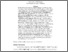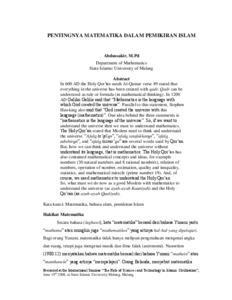# Pentingnya matematika dalam pemikiran Islam

Abdussakir, Abdussakir (2008) Pentingnya matematika dalam pemikiran Islam. Presented at International Seminar “The Role of Science and Technology in Islamic Civilization”, 19 Juli 2008, Fakultas Sains dan Teknologi Universitas Islam Negeri Maulana Malik Ibrahim Malang. (Unpublished)Preview
Text (Full text)
1751.pdf

## Abstract

In 600 AD the Holy Qor’an surah Al-Qamar verse 49 stated that everything in the universe has been created with qadr. Qadr can be understood as rule or formula (in mathematical thinking). In 1200 AD Galilio Galilie said that “Mathematics is the language with which God created the universe”. Parallel to this statement, Stephen Hawking also said that “God created the universe with this language (mathematics)”. One idea behind the three statements is “mathematics is the language of the universe”. So, if we want to understand the universe then we must to understand mathematics. The Holy Qur’an stated that Moslem need to think and understand the universe. “Afala ta’qilun”, “afala tatafakkarun”, “afala tubshirun”, and “afala tasma’un” are several words used by Qur’an. But, how we can think and understand the universe without understand its language, that is mathematics. The Holy Qur’an has also contained mathematical concepts and ideas, for example numbers (30 natural numbers and 8 rational numbers), relation of numbers, operation of number, estimation, quality and inequality, statistics, and mathematical miracle (prime number 19). And, of course, we need mathematics to understand the Holy Qur’an. So, what must we do now as a good Moslem with mathematics to understand the universe (as ayah-ayah Kauniyah) and the Holy Qur'an (as ayah-ayah Qualiyah)

Item Type: Seminar and Workshop 01 MATHEMATICAL SCIENCES > 0199 Other Mathematical Sciences > 019999 Mathematical Sciences not elsewhere classified Faculty of Mathematics and Sciences > Department of Mathematics Abdussakir Abdussakir 03 Apr 2017 23:00View Item